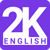# Latest Elitmus Aptitude Question SOLUTION: if v,w,x,y,z are non negative intergers each leass than 11,then how many distinct combinations are possible of(v,w,x,y,z) satisfy v(11^4) +w(11^3)+ x(11^2)+ y(11) +z=151001Launched an English app featuring 2000 mostly asked english words in all Competitive Exams. #2kenglish Download Now, It's Free